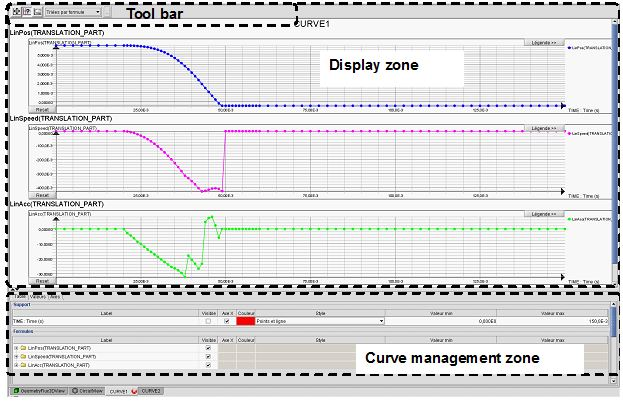# 2D Curve: environment (2D curve sheet)

## Environment of a 2D Curve

A 2D curve has got its own environment. The illustration below permits to identify each of the zones of the 2D curve sheet.The curves administration zone is subdivided into three tabs. The functions presented in each of the tabs are given in the table below.

Proposition name Tab Function
(Representation / Properties / control panel/ Quantities) Table
• Choice of quantities represented in the tracing zone
• Choice of quantity on abscissa
• Choice of quantities turned visible on ordinate
• Options for the graphic representation: color, style
Table of values/ List of calculated values / ... Values
• List of calculated values (table of values)
• Export of calculated values
Axes (scales) Axes
• Choice of the type of scale associated to the axes (lin, log)
• Choice of extreme (= numerical framing)

## Display zone

The display zone is the zone reserved to the various graphs of the elementary curves.

## Tool bar

The tool bar proposes the following tools:

• For value display (cursors)
• For framing (zoom, translation)
• For superposition of the elementary curves